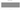\$

Eastern Caribbean Dollar

East Caribbean dollar

how has the value of the currency changed in the last year?USD 1 = XCD 2.7026

How much is10 US dollars worth inEast Caribbean dollars?
At the current exchange rate, 10 US dollars is worth 27.03 East Caribbean dollars
How much is50 US dollars worth inEast Caribbean dollars?
At the current exchange rate, 50 US dollars is worth 135.13 East Caribbean dollars
How much is100 US dollars worth inEast Caribbean dollars?
At the current exchange rate, 100 US dollars is worth 270.26 East Caribbean dollars
How much is500 US dollars worth inEast Caribbean dollars?
At the current exchange rate, 500 US dollars is worth 1,351.28 East Caribbean dollars
How much is2,000 US dollars worth inEast Caribbean dollars?
At the current exchange rate, 2,000 US dollars is worth 5,405.1 East Caribbean dollars
How much is10 US dollars worth inEast Caribbean dollars?
At the current exchange rate, 10 US dollars is worth 27.03 East Caribbean dollars
How much is50 US dollars worth inEast Caribbean dollars?
At the current exchange rate, 50 US dollars is worth 135.13 East Caribbean dollars
How much is100 US dollars worth inEast Caribbean dollars?
At the current exchange rate, 100 US dollars is worth 270.26 East Caribbean dollars
How much is500 US dollars worth inEast Caribbean dollars?
At the current exchange rate, 500 US dollars is worth 1,351.28 East Caribbean dollars
How much is2,000 US dollars worth inEast Caribbean dollars?
At the current exchange rate, 2,000 US dollars is worth 5,405.1 East Caribbean dollars